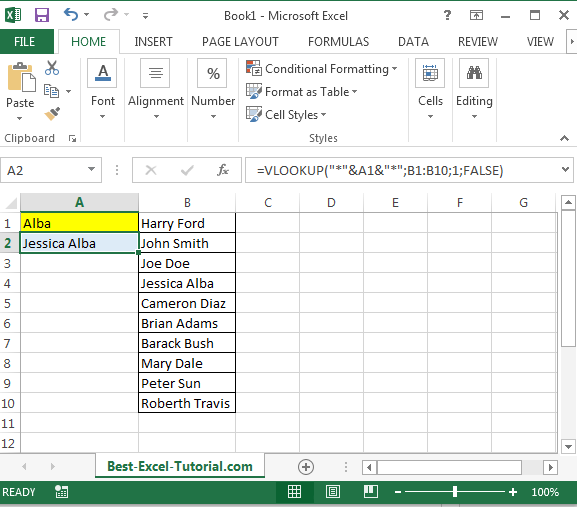#### How to do a Vlookup Using Text

In this Excel tutorial, you learn how to vlookup using string of text. This is a simple trick you will understand and use many times in Excel.

## Vlookup text formula

In Microsoft Excel, the VLOOKUP function can be used to search for a specific value in a table of data and return a related value from the same row. When using the VLOOKUP function with text values, you need to ensure that the data in the table is correctly formatted, and that the search criteria is correctly specified. For this, just use a vlookup with such a syntax:

=VLOOKUP(“*”&A1&”*”,B1:B10,1,FALSE)

where

“*” – some string of text

& – connection

A1 – here you have a string of text you are looking for in the array

& – again connection of formula

“*” – again some string of text

FALSE – that is kind of important, because you are looking for exact match here

## Vlookup text example

Here is an example of Vlookup used with text in Excel.

You have a column of first names and family names. You want to find out the name of some person, but you only remember their family name.